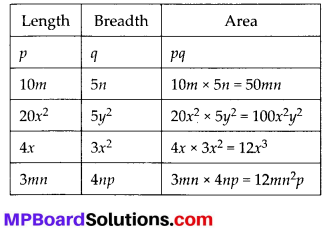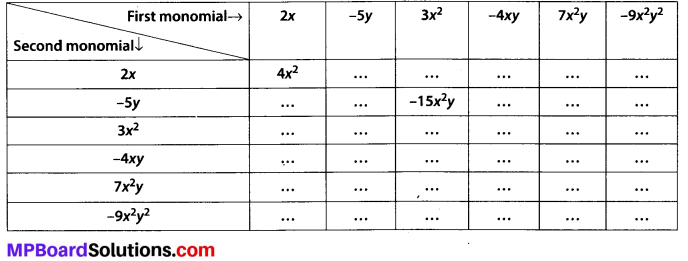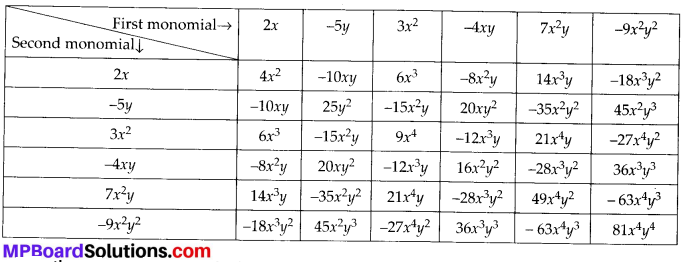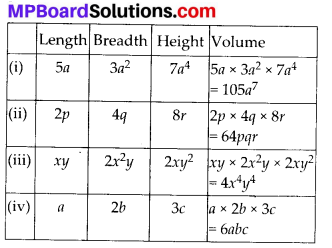# MP Board Class 8th Maths Solutions Chapter 9 Algebraic Expressions and Identities Ex 9.2

## MP Board Class 8th Maths Solutions Chapter 9 Algebraic Expressions and Identities Ex 9.2

Question 1.
Find the product of the following pairs of monomials.
(i) 4, 7p
(ii) -4p, 7p
(iii) -4p, 7pq
(iv) 4p3, -3p
(v) 4p, 0
Solution:
(i) 4 × 7p = 28p
(ii) -4p × 7p = -28p
(iii) -4p × 7pq = -28p
(iv) 4p3 × -3p = -12p4
(v) 4p × 0 = 0

Question 2.
Find the areas of rectangles with the following pairs of monomials as their lengths and breadths respectively.
(p, q); d (10m, 5n); (20x2, 5y2); (4x, 3x2); (3mn, 4np)
Solution:Question 3.
Complete the table of products.Solution:Question 4.
Obtain the volume of rectangular boxes with the following length, breadth and height respectively.
(i) 5a, 3a2, 7a4
(ii) 2p, 4q, 8r
(iii) xy, 2x2y, 2xy2
(iv) a, 2b, 3c
Solution:Question 5.
Obtain the product of
(i) xy, yz, zx
(ii) a, -a2, a2
(iii) 2, 4y, 8y2, 16y3
(iv) a, 2b, 3c. 6abc
(v) m, – mn, mnp
Solution:
(i) xy × yz × zx = x2y2z2
(ii) a × (-a2) × a3 = – a6
(iii) 2 × 4y × 8y2 × 16y3 = 1024y6
(iv) a × 2b × 3c × 6abc = 36a2b2c2
(v) m × (- mn) × mnp = – m3n2p